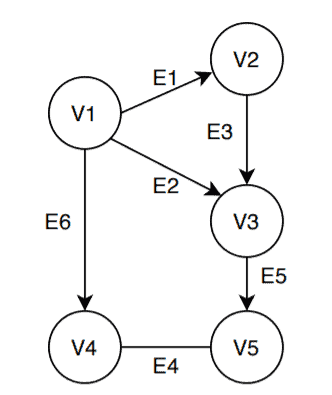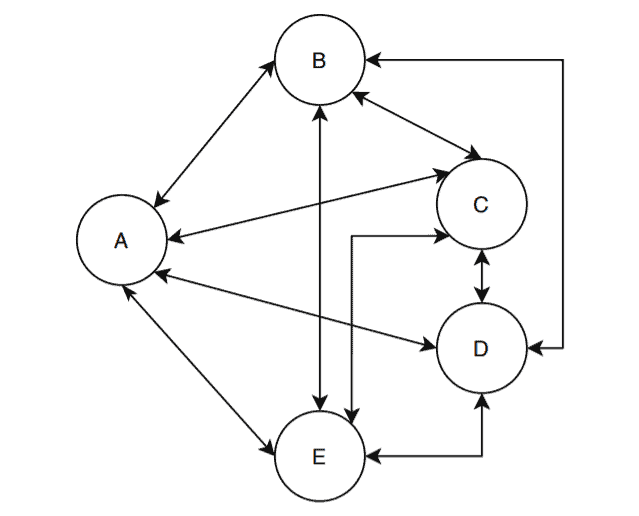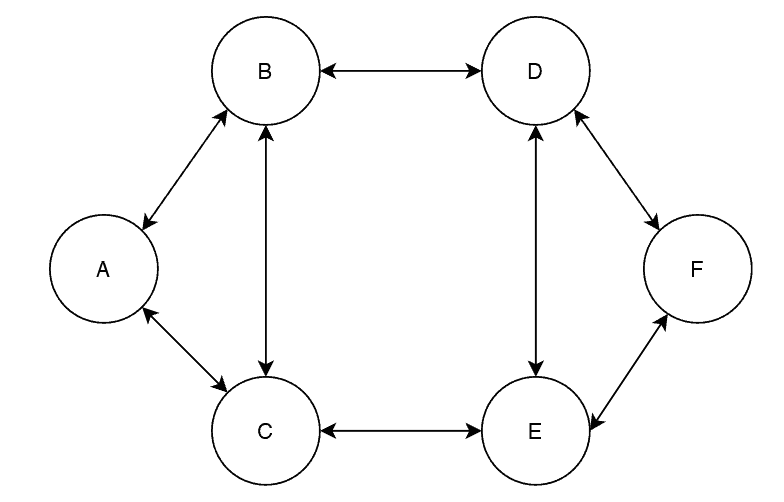## 1. Overview

In this tutorial, we’ll discuss how to calculate the maximum number of edges in a directed graph.

## 2. A Simple Definition of Directed Graph

In graph theory, graphs can be categorized generally as a directed or an undirected graph. In this section, we’ll focus our discussion on a directed graph.

Let’s start with a simple definition. A graphis a directed graph if all the edges in the graphhave direction. The vertices and edges inshould be connected, and all the edges are directed from one specific vertex to another.

The main difference between a directed and an undirected graph is reachability. Let’s explain this statement with an example:We’ve taken a graph. The vertex setcontains five vertices:. The edge setofcontains six edges:.

Now as we discussed, in a directed graph all the edges have a specific direction. For example, edgecan only go from vertexto. Unlike an undirected graph, now we can’t reach the vertexfromvia the edge.

Hence in a directed graph, reachability is limited and a user can specify the directions of the edges as per the requirement.

## 3. When a Directed Graph Contains Maximum Edges?

In this section, we’ll discuss some conditions that a directed graph needs to hold in order to contain the maximum number of edges.

Firstly, there should be at most one edge from a specific vertex to another vertex. This ensures all the vertices are connected and hence the graph contains the maximum number of edges. In short, a directed graph needs to be a complete graph in order to contain the maximum number of edges.

In graph theory, there are many variants of a directed graph. To make it simple, we’re considering a standard directed graph. So in our directed graph, we’ll not consider any self-loops or parallel edges.

## 4. A General Formula for Maximum Edges Calculation

In this section, we’ll present a general formula to calculate the maximum number of edges that a directed graph can contain.

Let’s assume an undirected graph withvertices. Further, we’re also assuming that the graph has a maximum number of edges. In such a case, from the starting vertex, we can drawedges in the graph. Continuing this way, from the next vertex we can drawedges.

Hence the maximum number of edges in an undirected graph is:Now, in an undirected graph, all the edges are bidirectional. We can convert an undirected graph into a directed graph by replacing each edge with two directed edges. Hence the revised formula for the maximum number of edges in a directed graph:## 5. An Example

In this section, we’ll take some directed graph and calculate the maximum number of edges according to the formula we derived:Now, we already discussed some conditions and assumptions for a directed graph such that it contains the maximum number of edges. Let’s verify first whether this graph contains the maximum number of edges or not.

First, let’s check if it is a complete directed graph or not. In a complete directed graph, all the vertices are reachable from one another. In the above graph, we can see all the vertices are reachable from one another.

Secondly, in our directed graph, there shouldn’t be any parallel edges or self-loop. Our example directed graph satisfies this condition too. Now let’s proceed with the edge calculation. Note that each edge here is bidirectional. Hence, each edge is counted as two independent directed edges.

The maximum number of edges =and the above graph has all the edges it can contain.

Let’s take another graph:Does this graph contain the maximum number of edges? Let’s check.

To verify this, we need to check if all the vertices can reach from one another. If we take a deep loop in the graph, we can see a lot of vertices can’t reach each other via a single edge.

Therefore, we can conclude that the given directed graph doesn’t contain the maximum number of edges. According to our formula, this graph has the capacity to contain maximum ofedges. But the graph has 16 edges in this example.

## 6. Conclusion

In this tutorial, we’ve discussed how to calculate the maximum number of edges in a directed graph.

We’ve presented a general formula for calculating the maximum number of edges in a directed graph and verified our formula with the help of a couple of examples.